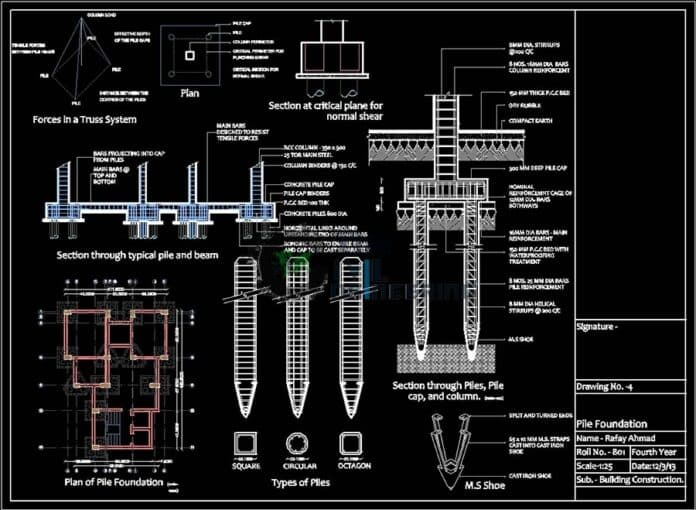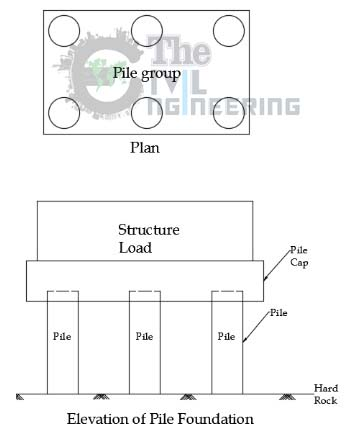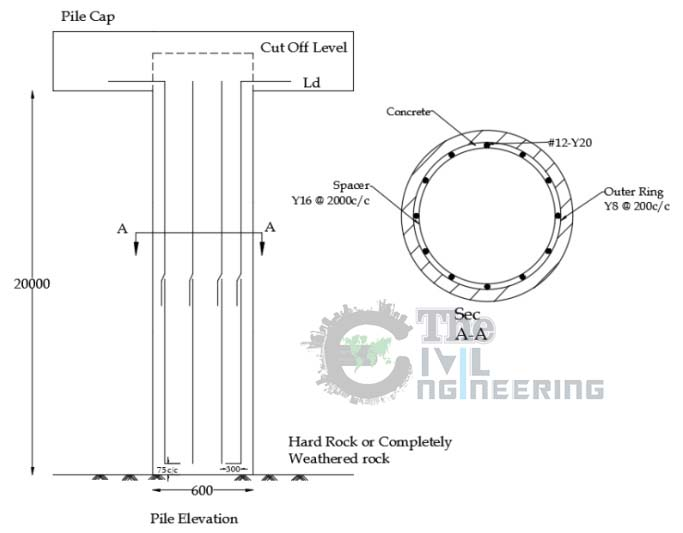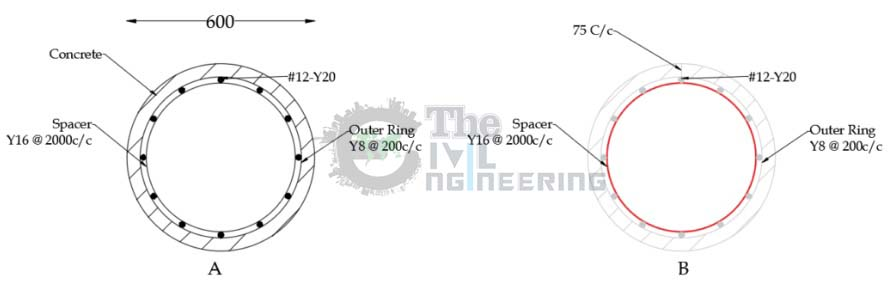# How to Make Bar Bending Schedule for Pile Foundation Reinforcement | BBS for Pile | Pile Ring Calculation Formula | Spiral Length Calculation Formula | Pile Helical Reinforcement Formula | BBS of Pile Cap:

## Pile Foundation Basics

Pile foundations are used in the below situations where the deep foundation is required.

• The Soil is highly compressible and too weak to support the load so we have to reach the hard strata
• The structure has horizontal forces which occur in skyscrapers (wind force)
• Uplifting Force by the excess water table.
• Presence of Expansive soils where soil constantly swells and shrinks like below

## Pile Foundation Diagram

Be familiar with typical pile foundation diagram.

It consists of

• Structure
• Pile Cap (supported by number of piles)## Bar Bending Schedule for Pile Foundation Reinforcement:

We have omitted the pile cap part since is a simple rectangular concrete slab which can be calculated by rectangular volume formula (L X B X h)

As you see in the above diagram

• The pile column should be tied by inner spacer ring and outer helical or spiral ring.
• The Anchorage bar will be bend into the column at bottom
• The Development length is provided outside at top of the column (which will be later inserted into Pile Cap)Bar Bending Schedule for Pile Foundation Reinforcement, BBS for Pile, Pile Ring Calculation Formula, Spiral Length Calculation Formula, Pile Helical Reinforcement Formula, BBS of Pile Cap

From the diagram,

• Height of the pile – 20m or 20000 mm
• Diameter of Pile – 600 mm
• Clear Cover of the pile – 75mm
• Column has 12 numbers of 12mm dia bars
• Inner Spacer Ring (circular ties) – 16mm @ 2000 mm C/C
• Outer Helical Ring – 8mm @ 200mm C/C
• Development Length (Ld) – 40d
• Bottom anchorage length – 300 mm

The Pile has three sections

1. Vertical bar
2. Inner Spacer Ring
3. Outer Helical Ring

### Step 1 – Calculate the Length of Vertical Bar:

Cutting length of vertical bar = Pile anchorage length Bottom + Height of Pile + Development Length Upper + Lap Length(50d) – Clear Cover bottom

#### Formula Explanation:

• As you know from the BBS for Column post, Each rod will be 12.2 m or 39 Feet length. So we have to lap additional bars in order to meet our requirement of 20 m height. That’s why we have added lap length (40d). Always tie the bars at middle, not at upper or bottom where the stress is high

Back to Formula,

Cutting length of vertical bar = Pile anchorage length Bottom + Height of Pile + Development Length Upper + Lap Length(50d) – Clear Cover bottom

= 300mm + 20000 mm + 40d + 50d – 75mm = 300mm + 20000 mm + (40X12) + (50X12) -75mm

= 21.30 m or 21305mm

Total Length of Vertical bar = 21.30 m

### Step 2 – Calculation of Number of Inner Spacer Ring & Length of Each Ring:

• Number of Inner Ring = ( Length of Pile / Spacing )+ 1 = (20000 mm/2000 mm) + 1 = 11 Numbers

Length of Each Ring,

Since it is circular figure we have to find the circumference of ring

• Length of Inner spacer ring = Circumference of Inner Ring  = 2丌r (where R – Radius)

Now we need to know the radius of inner spacer ring

We already know the diameter of the pile(600mm) so it is easy to find the diameter of inner ring and the radiusDiameter of Inner Ring = Diameter of Pile – Clear Cover – Dia of outer spiral ring – Diameter of vertical bar

= 600mm – 75mm – 8mm – 12mm

Diameter of Inner Ring = 505 mm

Radius of Circle (R) = D/2 Therefore radius of Inner ring = 505mm/2 = 252.5 or 253 mm

Length of Inner Spacer Ring = Circumference of Inner Ring =  2丌r (where R – Radius) = 2 X 3.14 X 253mm

Length of Inner Spacer Ring = 1588 mm or 1.59 m

### Step 3 – Calculation of Number of Outer Helical Ring & Length of Each Ring:

From the Diagram,

• Length of Pile – 20 m or 20000 mm
• Diameter of Pile – 600 mm
• Spacing of Outer Helical Ring – 200 mm
• Diameter of Helical Ring – 8mm

Again as above in order to find the length of the helical outer ring we have to find the circumference of the ring

• Length of Inner spacer ring = Circumference of Inner Ring  = 2丌r (where R – Radius)

We already know the diameter of the pile(600mm) so it is easy to find the diameter of inner ring and the radius

Diameter of outer spiral ring = Diameter of Pile – Clear Cover  = 600mm – 75mm = 525mm

Therefore Radius of spiral ring (R) = D/2 Therefore radius of Inner ring = 525mm/2 = 262.5 or 263 mm

Length of One spiral ring  = Circumference of Inner Ring  = 2丌r (where R – Radius) = 2 x 3.14 X 263mm

Length of one spiral ring = 1652 mm or 1.65 m

Now we have to find how many number of spiral ring we need

Required Number of Spiral Rings = ( Length of Pile / Spacing ) + 1 = (20000 / 200) + 1 = 101 spirals or numbers